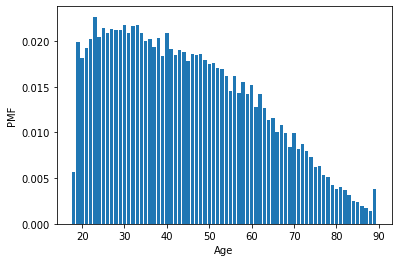Probability mass functions

import pandas as pd
import numpy as np
import matplotlib.pyplot as plt
import seaborn as sns
from empiricaldist import Pmf, Cdf
from scipy.stats import norm
year age cohort sex race educ realinc gunlaw grass wtssall
0 1972 26.0 1946.0 1 1 18.0 13537.0 2.0 NaN 0.8893
1 1972 38.0 1934.0 2 1 12.0 18951.0 1.0 NaN 0.4446
2 1972 57.0 1915.0 1 1 12.0 30458.0 1.0 NaN 1.3339
3 1972 61.0 1911.0 2 1 14.0 37226.0 1.0 NaN 0.8893
4 1972 59.0 1913.0 1 1 12.0 30458.0 2.0 NaN 0.8893

Make a PMF

year = gss['year']
# Compute the PMF for year
pmf_year = Pmf.from_seq(year, normalize=False)

# Print the result
print(pmf_year)
1972    1613
1973    1504
1974    1484
1975    1490
1976    1499
1977    1530
1978    1532
1980    1468
1982    1860
1983    1599
1984    1473
1985    1534
1986    1470
1987    1819
1988    1481
1989    1537
1990    1372
1991    1517
1993    1606
1994    2992
1996    2904
1998    2832
2000    2817
2002    2765
2004    2812
2006    4510
2008    2023
2010    2044
2012    1974
2014    2538
2016    2867
Name: year, dtype: int64

Plot a PMF

age = gss['age']

# Make a PMF of age
pmf_age = Pmf.from_seq(age)

# Plot the PMF
pmf_age.bar()

# Label the axes
plt.xlabel('Age')
plt.ylabel('PMF')
Text(0, 0.5, 'PMF')Cumulative distribution functions

Make a CDF

age = gss['age']

# Compute the CDF of age
cdf_age = Cdf.from_seq(age)

# Calculate the CDF of 30
print(cdf_age(30))
print(1 - cdf_age(30))
0.2539137136526389
0.7460862863473612

Compute IQR

income = gss['realinc']

cdf_income = Cdf.from_seq(income)
percentile_75th = cdf_income.inverse(0.75)

# Calculate the 25th percentile
percentile_25th = cdf_income.inverse(0.25)

# Calculate the interquartile range
iqr = percentile_75th - percentile_25th

# Print the interquartile range
print(iqr)
29676.0

Plot a CDF

cdf_income.plot()

# Label the axes
plt.xlabel('Income (1986 USD)')
plt.ylabel('CDF')
Text(0, 0.5, 'CDF')Comparing distribution

Extract education levels

educ = gss['educ']

# Bachelor`s degree
bach = (educ >= 16)

# Associate degree
assc = ((educ >= 14) & (educ < 16))

# High school (12 or fewer years of education)
high = (educ <= 12)
print(high.mean())
0.5308807991547402

Plot income CDFs

income = gss['realinc']

# Plot the CDFs
Cdf.from_seq(income[high]).plot(label='High school')
Cdf.from_seq(income[assc]).plot(label='Associate')
Cdf.from_seq(income[bach]).plot(label='Bachelor')

# Label the axes
plt.xlabel('Income (1986 USD)')
plt.ylabel('CDF')
plt.legend()
plt.savefig('../images/income-cdf.png')Modeling distributions

Distribution of income

log_income = np.log10(income)

# Compute mean and standard deviation
mean = np.mean(log_income)
std = np.std(log_income)
print(mean, std)

# Make a norm object
dist = norm(mean, std)
4.371148677933652 0.4290043733010077

Comparing CDFs

xs = np.linspace(2, 5.5)
ys = dist.cdf(xs)

# Plot the model CDF
plt.plot(xs, ys, color='gray')

# Create and plot the Cdf of log_income
Cdf.from_seq(log_income).plot()

# Label the axes
plt.xlabel('log10 of realinc')
plt.ylabel('CDF')
Text(0, 0.5, 'CDF')xs = np.linspace(2, 5.5)
ys = dist.pdf(xs)

# Plot the model CDF
plt.plot(xs, ys, color='gray')

# Plot the data KDE
sns.kdeplot(log_income)

# Label the axes
plt.xlabel('log10 of realinc')
plt.ylabel('CDF')
Text(0, 0.5, 'CDF')